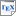[BCKL19] Luca Bortolussi, Luca Cardelli, Marta Kwiatkowska and Luca Laurenti. Central Limit Model Checking. ACM Transactions on Computational Logic (TOCL), 20(4), pages 1-35, ACM New York, NY, USA. 2019. [pdf] [bib] https://doi.org/10.1145/3331452 Downloads:pdf (2.87 MB)bib Links: [Google] [Google Scholar] [CiteSeer] Available from: https://doi.org/10.1145/3331452 Abstract. We consider probabilistic model checking for continuous-time Markov chains (CTMCs) induced from Stochastic Reaction Networks against a fragment of Continuous Stochastic Logic (CSL) extended with reward operators. Classical numerical algorithms for CSL model checking based on uniformisation are limited to finite CTMCs and suffer from exponential growth of the state space with respect to the number of species. However, approximate techniques such as mean-field approximations and simulations combined with statistical inference are more scalable but can be time-consuming and do not support the full expressiveness of CSL. In this article, we employ a continuous space approximation of the CTMC in terms of a Gaussian process based on the Central Limit Approximation, also known as the Linear Noise Approximation, whose solution requires solving a number of differential equations that is quadratic in the number of species and independent of the population size. We then develop efficient and scalable approximate model checking algorithms on the resulting Gaussian process, where we restrict the target regions for probabilistic reachability to convex polytopes. This allows us to derive an abstraction in terms of a time-inhomogeneous discrete-time Markov chain (DTMC), whose dimension is independent of the number of species, on which model checking is performed. Using results from probability theory, we prove the convergence in distribution of our algorithms to the corresponding measures on the original CTMC. We implement the techniques and, on a set of examples, demonstrate that they allow us to overcome the state space explosion problem, while still correctly characterizing the stochastic behaviour of the system. Our methods can be used for formal analysis of a wide range of distributed stochastic systems, including biochemical systems, sensor networks, and population protocols.